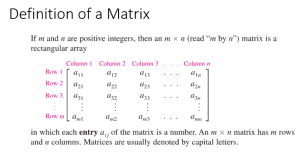Matrices and Determinants

//Matrices and Determinants

Matrices and Systems of Equations

You can use a matrix to solve a system of equations. There are many ways to do so. One of them involves finding the augmented matrix and performing row operations on that matrix using the Gauss Elimination method and the Gauss-Jordan Elimination method. Both will be explored in this chapter among other methods.Solve systems of three equations using matrices.

Matrices and Systems of Equations Section 8-1 notes

Operations with Matrices

Add, subtract, and multiply matrices by scalars.

Multiply Matrices

Operations with Matrices Section 8-2 notes

Inverses of Matrices

The Inverse of a Square Matrix Section 8-3 Notes

8-1 to 8-3 Review

8-1 to 8-3 Review Key

By | 2017-11-13T22:01:57+00:00 November 2nd, 2015|Uncategorized|0 Comments